Effective at SESYNC's closure in December 2022, this page is no longer maintained. The information may be out of date or inaccurate.

# Remote Sensing & Classification

## Hurricane Inundation

Note: This lesson is in beta status! It may have issues that have not been addressed.

Handouts for this lesson need to be saved on your computer. Download and unzip this material into the directory (a.k.a. folder) where you plan to work.

Remote Sensing allows for fast generation of maps before and after events. In this exercise, you will map flooding using machine learning methods and MODIS reflectance data (MOD09). Hurricanes can strongly impact vegetation and human settlements. Hurricane Rita made landfall on September 24, 2005 in Louisiana near the border with Texas. In addition to the rainfall, the hurricane was accompanied by a storm surge that generated flooding and a large amount of damage to ground infrastructure and vegetation. As a geospatial analyst, you will experiment with different classifiers for rapid mapping and examine their performance with training and testing data. All work must be repeatable and documented in a scripting environment to be shared with your team.

## Inputs

• FEMA flood map (stored as vector data)
• MOD09 reflectance image after Hurricane Rita
• Vector polygons of ground truth (for training and testing classifiers)
• NLCD 2006 land cover aggregated at 1km

## Outputs

• classified map using rpart classifier
• classified map using svm classifier
• confusion matrices and overall accuracies by classifiers

## Tools/Functions

• raster::brick
• raster::writeRaster
• raster::subset
• raster::extract
• raster::predict
• raster algebra: “*”,” /”,”+”,”-“
• rasterVis::levelplot
• rasterVis::histogram

## Part I: Read and map flooding from RITA hurricane

Explore the datasets provided. Use ground truth vector datasets and extract values for pixels for the raster stack containing the original MOD09 bands and indices. Visualize ground truth pixels in feature space and using boxplots.

Products:

• Scatterplot in feature space with the three ground classes.
• Boxplots of values for MNDWI indices.

## Part II: Split data into training and testing datasets

Assessment of classifications require splitting of the ground truth data into training and testing samples. The training data is used in the fitting of the model while the testing dataset is used accuracy assessment. Using the data provided randomly select 30% ground truth data for testing.

Products:

• Training and testing datasets at 30%. Save these into shapefiles.

Be able to answer the following question:

• What is the frequency of observations in each class for training and testing data?

## Part III: Generate classification using classification tree (CART) and support vector machine (SVM)

You will use MOD09 reflectance bands to generate a classification of three classes (“vegetation”, “wetland”, “water”). Fit a model with the training data for each method and generate a classification on a raster using the “predict” command.

RPART: It is based on CART and generates classification by splitting data points into branches successively and generating classified pixels. The rules generated from the tree can then be applied to the full raster image to produce a prediction.

SVM: Support Vector Machine generate hyperplanes that maximize separabiltiy between classes.These hyperplanes boundaries are used in the classification process and generate predictions.

Products:

• Model object for rpart with a predicted raster.
• Model object for svm with a predicted raster

## Part IV: Assessment and comparison of model performance

To validate the model, we use the testing data and generate new predictions for rpart and SVM. We compare the predictions with the original testing information.

Overall accuracy: total number of correctly classified pixels for all classes combined over the total number of pixels.

Producer accuracy: is the fraction of correctly classified pixels with regard to all pixels of the ground truth class.

Products:

• Overall accuracy and producer accuracy metrics.
• Confusion matrix for rpart and SVM.

Be able to answer the following question:

• What classifier had the best overall accuracy?

Using the analyses performed above, and datasets provided answer to the following questions:

• Modify the splitting of training and testing by using 40% and then 50% for testing. Compare predicted maps with previous results.
• Generate confusion matrices for 40% and 50% testing.
• Generate a classification prediction using randomForest and compare it to svm and rpart with 30% holdout.

Top of Section

### Set up arguments and parameters

in_dir <- "data"

out_dir_suffix <- "rsc_lesson"
out_dir <- paste("output_", out_dir_suffix, sep = "")
dir.create(out_dir, showWarnings = FALSE)


Regional coordinate reference system taken from: http://spatialreference.org/ref/epsg/nad83-texas-state-mapping-system/proj4/

CRS_reg <- "+proj=lcc +lat_1=27.41666666666667 +lat_2=34.91666666666666 +lat_0=31.16666666666667 +lon_0=-100 +x_0=1000000 +y_0=1000000 +ellps=GRS80 +datum=NAD83 +units=m +no_defs"

file_format <- ".tif"

NA_flag_val <- -9999

out_suffix <- "raster_classification" # output suffix for the files and output folder


### Define input data file names

infile_RITA_reflectance_date2 <- "mosaiced_MOD09A1_A2005273__006_reflectance_masked_RITA_reg_1km.tif"

infile_reg_outline <- "new_strata_rita_10282017" # Region outline and FEMA zones

infile_modis_bands_information <- "df_modis_band_info.txt" # MOD09 bands information.

nlcd_2006_filename <- "nlcd_2006_RITA.tif" # NLCD2006 Land cover data aggregated at ~ 1km.


## Part I: Read and map flooding from RITA hurricane

### Display and explore the data

MOD09 raster image after hurricane Rita.

r_after <- brick(file.path(in_dir, infile_RITA_reflectance_date2))

> head(r_after)

      mosaiced_MOD09A1_A2005273__006_reflectance_masked_RITA_reg_1km.1
[1,]                                                       0.04558113
[2,]                                                       0.04460510
[3,]                                                       0.04348749
[4,]                                                       0.04507260
[5,]                                                       0.04715690
[6,]                                                       0.03485106
[7,]                                                       0.03807684
[8,]                                                       0.04214056
[9,]                                                       0.04512509
[10,]                                                       0.04687950
[11,]                                                       0.04639116
[12,]                                                       0.04591873
[13,]                                                       0.04590000
[14,]                                                       0.05590000
[15,]                                                       0.05367381
[16,]                                                       0.05220000
[17,]                                                       0.05146399
[18,]                                                       0.04496353
[19,]                                                       0.04380151
[20,]                                                       0.06080000
[1,]                                                        0.3027903
[2,]                                                        0.3043112
[3,]                                                        0.2998908
[4,]                                                        0.3017146
[5,]                                                        0.3239896
[6,]                                                        0.2683164
[7,]                                                        0.2991171
[8,]                                                        0.3176138
[9,]                                                        0.3125040
[10,]                                                        0.2979249
[11,]                                                        0.3101786
[12,]                                                        0.3076983
[13,]                                                        0.3076000
[14,]                                                        0.2953000
[15,]                                                        0.3113045
[16,]                                                        0.3219000
[17,]                                                        0.3242094
[18,]                                                        0.3254988
[19,]                                                        0.3138167
[20,]                                                        0.3098000
[1,]                                                       0.02379627
[2,]                                                       0.02354558
[3,]                                                       0.02374887
[4,]                                                       0.02426019
[5,]                                                       0.02511090
[6,]                                                       0.01941371
[7,]                                                       0.02170737
[8,]                                                       0.02348050
[9,]                                                       0.02387191
[10,]                                                       0.02560453
[11,]                                                       0.02765067
[12,]                                                       0.02635150
[13,]                                                       0.02630000
[14,]                                                       0.03030000
[15,]                                                       0.02999916
[16,]                                                       0.02980000
[17,]                                                       0.02756310
[18,]                                                       0.02447605
[19,]                                                       0.02306678
[20,]                                                       0.03420000
[1,]                                                       0.05476730
[2,]                                                       0.05586702
[3,]                                                       0.05381133
[4,]                                                       0.05503851
[5,]                                                       0.05962222
[6,]                                                       0.04450204
[7,]                                                       0.04927124
[8,]                                                       0.05364913
[9,]                                                       0.05487191
[10,]                                                       0.05564768
[11,]                                                       0.05650510
[12,]                                                       0.05544214
[13,]                                                       0.05540000
[14,]                                                       0.05870000
[15,]                                                       0.06014401
[16,]                                                       0.06110000
[17,]                                                       0.06231735
[18,]                                                       0.05556127
[19,]                                                       0.05338556
[20,]                                                       0.06660000
[1,]                                                        0.3318335
[2,]                                                        0.3138186
[3,]                                                        0.3123330
[4,]                                                        0.3193893
[5,]                                                        0.3417773
[6,]                                                        0.2855803
[7,]                                                        0.3055280
[8,]                                                        0.3266653
[9,]                                                        0.3330796
[10,]                                                        0.3139284
[11,]                                                        0.3352741
[12,]                                                        0.3151959
[13,]                                                        0.3144000
[14,]                                                        0.3485000
[15,]                                                        0.3409190
[16,]                                                        0.3359000
[17,]                                                        0.3346323
[18,]                                                        0.3365578
[19,]                                                        0.3272987
[20,]                                                        0.3235000
[1,]                                                        0.1902746
[2,]                                                        0.1842244
[3,]                                                        0.1855501
[4,]                                                        0.1913280
[5,]                                                        0.1930779
[6,]                                                        0.1609111
[7,]                                                        0.1696934
[8,]                                                        0.1781321
[9,]                                                        0.1907084
[10,]                                                        0.1913635
[11,]                                                        0.1840112
[12,]                                                        0.1886174
[13,]                                                        0.1888000
[14,]                                                        0.2295000
[15,]                                                        0.2199936
[16,]                                                        0.2137000
[17,]                                                        0.2036836
[18,]                                                        0.1829669
[19,]                                                        0.1818172
[20,]                                                        0.2122000
[1,]                                                       0.07314926
[2,]                                                       0.06792310
[3,]                                                       0.07248760
[4,]                                                       0.07654412
[5,]                                                       0.07032830
[6,]                                                       0.05962812
[7,]                                                       0.06153552
[8,]                                                       0.06410249
[9,]                                                       0.07345820
[10,]                                                       0.07704768
[11,]                                                       0.06562383
[12,]                                                       0.07223780
[13,]                                                       0.07250001
[14,]                                                       0.10140000
[15,]                                                       0.09135209
[16,]                                                       0.08470000
[17,]                                                       0.07624704
[18,]                                                       0.06806806
[19,]                                                       0.06944025
[20,]                                                       0.09370000

> inMemory(r_after) # check if raster in memory

 FALSE


df_modis_band_info <- read.table(file.path(in_dir, infile_modis_bands_information),
sep=",", stringsAsFactors = F)

> df_modis_band_info

  band_name band_number start_wlength end_wlength
1       Red           3           620         670
2       NIR           4           841         876
3      Blue           1           459         479
4     Green           2           545         565
5     SWIR1           5          1230        1250
6     SWIR2           6          1628        1652
7     SWIR3           7          2105        2155

names(r_after) <- df_modis_band_info$band_name  > names(r_after)   "Red" "NIR" "Blue" "Green" "SWIR1" "SWIR2" "SWIR3"  Define bands of interest: Use subset instead of$ if you want to wrap code into function

raft_gr <- subset(r_after, "Green")
raft_SWIR <- subset(r_after, "SWIR2")
raft_NIR <- subset(r_after, "NIR")
raft_rd <- subset(r_after, "Red")


Manipulate bands of interest

r_after_MNDWI <- (raft_gr - raft_SWIR) / (raft_gr + raft_SWIR)

plot(r_after_MNDWI, zlim = c(-1,1))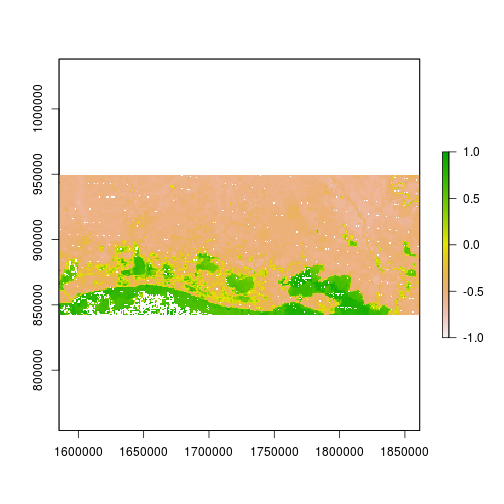r_after_NDVI <- (raft_NIR - raft_rd) / (raft_NIR + raft_rd)

plot(r_after_NDVI)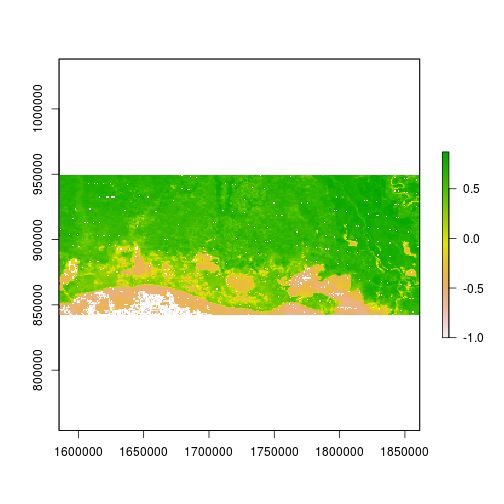> inMemory(r_after_MNDWI) # check if raster in memory

 TRUE

> inMemory(r_after_NDVI)

 TRUE


Write out rasters

> dataType(r_after_NDVI)

 "FLT4S"

data_type_str <- dataType(r_after_NDVI)

NAvalue(r_after_MNDWI) <- NA_flag_val # set NA value
out_filename_mn <- file.path(out_dir, paste0("mndwi_post_Rita","_", out_suffix, file_format))
writeRaster(r_after_MNDWI, filename = out_filename_mn, datatype = data_type_str,
overwrite = T)

NAvalue(r_after_NDVI) <- NA_flag_val
out_filename_nd <- file.path(out_dir, paste0("ndvi_post_Rita","_", out_suffix, file_format))
writeRaster(r_after_NDVI, filename = out_filename_nd, datatype = data_type_str,
overwrite = T)


Remove rasters from memory

rm(r_after_MNDWI)
rm(r_after_NDVI)


Can view the .tif images written out in photo viewer.

r_after_MNDWI <- raster(out_filename_mn)
r_after_NDVI <- raster(out_filename_nd)

> inMemory(r_after_MNDWI) ; inMemory(r_after_NDVI)

 FALSE

 FALSE


### Groundtruthing data

Three levels of flooding (water content) determined via external grountruthing. Class 1: vegetation and other (small water fraction) Class 2: Flooded vegetation Class 3: Flooded area, or water (lake etc)

Read in a polygon for each class:

class1_data_sf <- st_read(file.path(in_dir, "class1_sites"))

Reading layer class1_sites' from data source /nfs/public-data/training/class1_sites' using driver ESRI Shapefile'
Simple feature collection with 6 features and 2 fields
geometry type:  POLYGON
dimension:      XY
bbox:           xmin: 1588619 ymin: 859495.9 xmax: 1830803 ymax: 941484.1
CRS:            NA

class2_data_sf <- st_read(file.path(in_dir, "class2_sites"))

Reading layer class2_sites' from data source /nfs/public-data/training/class2_sites' using driver ESRI Shapefile'
Simple feature collection with 8 features and 2 fields
geometry type:  MULTIPOLYGON
dimension:      XY
bbox:           xmin: 1605433 ymin: 848843.8 xmax: 1794265 ymax: 884027.8
CRS:            NA

class3_data_sf <- st_read(file.path(in_dir, "class3_sites"))

Reading layer class3_sites' from data source /nfs/public-data/training/class3_sites' using driver ESRI Shapefile'
Simple feature collection with 9 features and 2 fields
geometry type:  POLYGON
dimension:      XY
bbox:           xmin: 1587649 ymin: 845271.3 xmax: 1777404 ymax: 891073.1
CRS:            NA

> class1_data_sf

Simple feature collection with 6 features and 2 fields
geometry type:  POLYGON
dimension:      XY
bbox:           xmin: 1588619 ymin: 859495.9 xmax: 1830803 ymax: 941484.1
CRS:            NA
record_ID class_ID                       geometry
1         1        1 POLYGON ((1610283 929863.7,...
2         2        1 POLYGON ((1755141 900167.2,...
3         3        1 POLYGON ((1688209 924699.1,...
4         4        1 POLYGON ((1821103 866597.2,...
5         5        1 POLYGON ((1806876 941161.3,...
6         6        1 POLYGON ((1588619 899198.8,...

> plot(r_after_MNDWI, zlim=c(-1,1))
> plot(class3_data_sf["class_ID"], add = TRUE, color = NA, border = "blue", lwd = 3)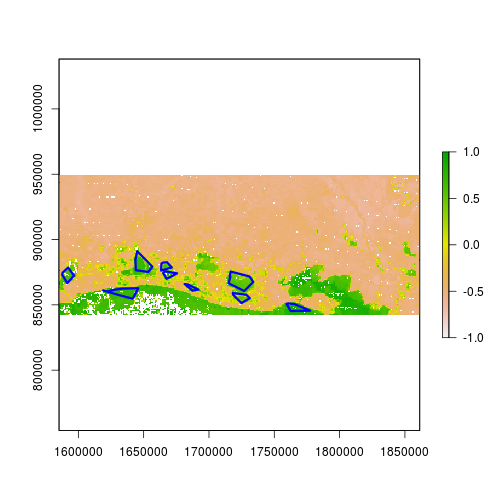Combine polygons; note they should be in the same projection system.

class_data_sf <- rbind(class1_data_sf, class2_data_sf, class3_data_sf)

class_data_sf$poly_ID <- 1:nrow(class_data_sf) # unique ID for each polygon  23 different polygons used as ground truth data. > nrow(class_data_sf)   23  Make new rasters and raster stack. r_x <- init(r_after, "x") # initializes a raster with coordinates x r_y <- init(r_after, "y") # initializes a raster with coordiates y r_stack <- stack(r_x, r_y, r_after, r_after_NDVI, r_after_MNDWI) names(r_stack) <- c("x", "y", "Red", "NIR", "Blue", "Green", "SWIR1", "SWIR2", "SWIR3", "NDVI", "MNDWI")  > str(r_stack, max.level = 2)  Formal class 'RasterStack' [package "raster"] with 11 slots ..@ filename: chr "" ..@ layers :List of 11 ..@ title : chr(0) ..@ extent :Formal class 'Extent' [package "raster"] with 4 slots ..@ rotated : logi FALSE ..@ rotation:Formal class '.Rotation' [package "raster"] with 2 slots ..@ ncols : int 298 ..@ nrows : int 116 ..@ crs :Formal class 'CRS' [package "sp"] with 1 slot ..@ history : list() ..@ z : list()  pixels_extracted_df <- extract(r_stack, class_data_sf, df=T)  > dim(pixels_extracted_df)   1547 12  We have 1547 pixels extracted. Sometimes useful to store data as a csv: have to remove geometry column. class_data_df <- class_data_sf st_geometry(class_data_df) <- NULL # This will coerce the sf object into a data.frame by removing the geometry  Make the dataframe used for classification. pixels_df <- merge(pixels_extracted_df, class_data_df, by.x="ID", by.y="poly_ID")  > head(pixels_df)   ID x y Red NIR Blue Green SWIR1 1 1 1615340 936279.2 0.05304649 0.3035397 0.02652384 0.05662384 0.3101271 2 1 1614413 935352.5 0.05782417 0.3295924 0.02751791 0.06305390 0.3453724 3 1 1615340 935352.5 0.05735013 0.3037102 0.02596963 0.05654212 0.3176476 4 1 1616266 935352.5 0.05805403 0.3081988 0.02711912 0.05750944 0.3229090 5 1 1617193 935352.5 0.04147716 0.3058677 0.01839499 0.05076184 0.3003513 6 1 1618120 935352.5 0.04209801 0.3089238 0.01832494 0.05200353 0.2943777 SWIR2 SWIR3 NDVI MNDWI record_ID class_ID 1 0.1936452 0.07864757 0.7024759 -0.5474963 1 1 2 0.2102322 0.08679578 0.7014884 -0.5385503 1 1 3 0.2073480 0.08732812 0.6823238 -0.5714722 1 1 4 0.2079238 0.08741243 0.6829839 -0.5666749 1 1 5 0.1692552 0.06590501 0.7611759 -0.5385644 1 1 6 0.1697936 0.06790353 0.7601402 -0.5310712 1 1  table(pixels_df$class_ID) # count by class of pixels ground truth data


1   2   3
508 285 754


### Examining data used for the classification

Define geographic range.

x_range <- range(pixels_df$Green, na.rm=T) y_range <- range(pixels_df$NIR, na.rm=T)


Let’s use a palette that reflects flooding or level of water.

col_palette <- c("green", "lightblue", "darkblue")


Many ways of looking at these data:

> plot(NIR~Green, xlim=x_range, ylim=y_range, cex=0.3, col=col_palette, subset(pixels_df, class_ID==1))
> points(NIR~Green, col=col_palette, cex=0.3, subset(pixels_df, class_ID==2))
> points(NIR~Green, col=col_palette, cex=0.3, subset(pixels_df, class_ID==3))
> names_vals <- c("vegetation","wetland","water")
> legend("topleft", legend=names_vals,
+        pt.cex=0.7, cex=0.7, col=col_palette,
+        pch=20, # add circle symbol to line
+        bty="n")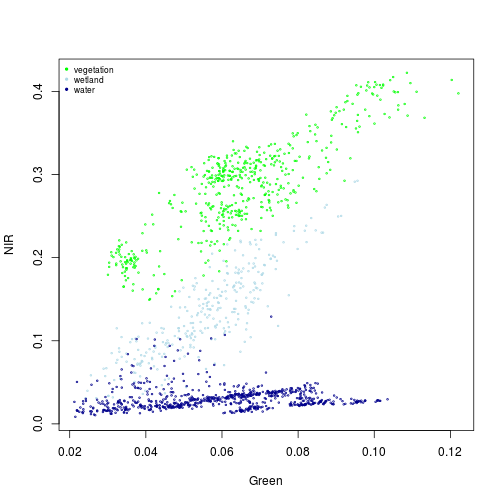plot(NDVI ~ MNDWI, xlim=c(-1,1), ylim=c(-1,1), cex=0.3, col=col_palette, subset(pixels_df, class_ID==1))
points(NDVI ~ MNDWI, cex=0.3, col=col_palette, subset(pixels_df, class_ID==2))
points(NDVI ~ MNDWI, cex=0.3, col=col_palette, subset(pixels_df, class_ID==3))
names_vals <- c("vegetation","wetland","water")
legend("topright", legend=names_vals,
pt.cex=0.7,cex=0.7, col=col_palette,
pch=20, # add circle symbol to line
bty="n")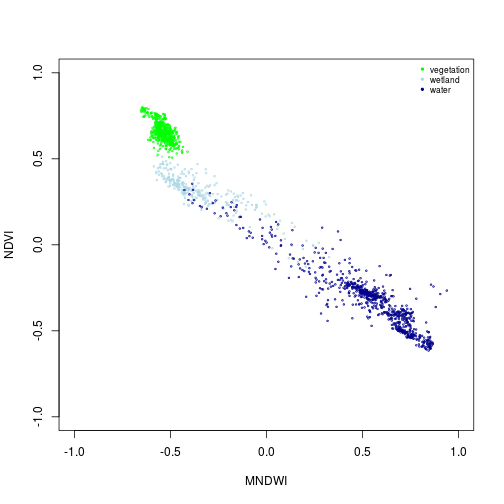rasterVis::histogram(r_after)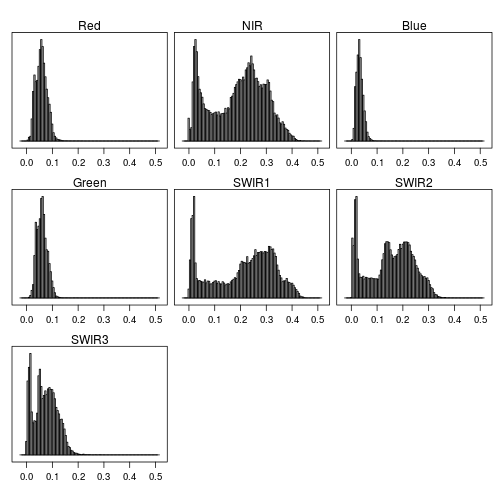pixels_df$class_ID <- factor(pixels_df$class_ID, levels = c(1,2,3), labels = names_vals)

boxplot(MNDWI~class_ID, pixels_df, xlab="category",
main="Boxplot for MNDWI per class")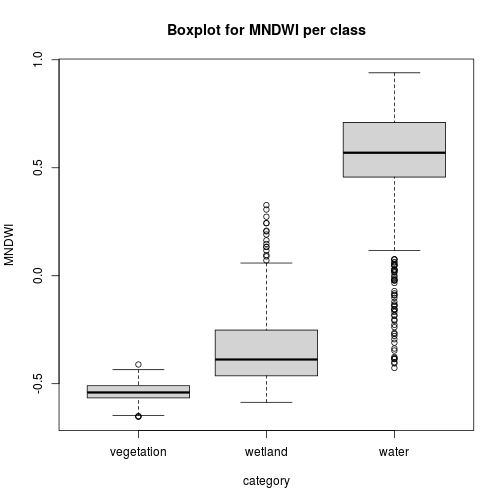## Part II: Split data into training and testing datasets

Let’s keep 30% of data for testing for each class, and set a fixed seed for reproducibility.

set.seed(100)
prop <- 0.3
table(pixels_df$class_ID)   vegetation wetland water 508 285 754  list_data_df <- vector("list", length=3) level_labels <- names_vals for(i in 1:3){ data_df <- subset(pixels_df, class_ID==level_labels[i]) data_df$pix_id <- 1:nrow(data_df)
indices <- as.vector(createDataPartition(data_df$pix_id, p=(1-prop), list=F)) data_df$training <- as.numeric(data_df$pix_id %in% indices) list_data_df[[i]] <- data_df } data_df <- do.call(rbind, list_data_df)  > dim(data_df)   1547 16  > head(data_df)   ID x y Red NIR Blue Green SWIR1 1 1 1615340 936279.2 0.05304649 0.3035397 0.02652384 0.05662384 0.3101271 2 1 1614413 935352.5 0.05782417 0.3295924 0.02751791 0.06305390 0.3453724 3 1 1615340 935352.5 0.05735013 0.3037102 0.02596963 0.05654212 0.3176476 4 1 1616266 935352.5 0.05805403 0.3081988 0.02711912 0.05750944 0.3229090 5 1 1617193 935352.5 0.04147716 0.3058677 0.01839499 0.05076184 0.3003513 6 1 1618120 935352.5 0.04209801 0.3089238 0.01832494 0.05200353 0.2943777 SWIR2 SWIR3 NDVI MNDWI record_ID class_ID pix_id 1 0.1936452 0.07864757 0.7024759 -0.5474963 1 vegetation 1 2 0.2102322 0.08679578 0.7014884 -0.5385503 1 vegetation 2 3 0.2073480 0.08732812 0.6823238 -0.5714722 1 vegetation 3 4 0.2079238 0.08741243 0.6829839 -0.5666749 1 vegetation 4 5 0.1692552 0.06590501 0.7611759 -0.5385644 1 vegetation 5 6 0.1697936 0.06790353 0.7601402 -0.5310712 1 vegetation 6 training 1 0 2 1 3 1 4 1 5 1 6 0  data_training <- subset(data_df, training==1)  ## Part III: Generate classification using classification tree (CART) and support vector machine (SVM) ### Classification and Regression Tree model (CART) Fit model using training data for CART. mod_rpart <- rpart(class_ID ~ Red + NIR + Blue + Green + SWIR1 + SWIR2 + SWIR3, method="class", data=data_training)  Plot the fitted classification tree. plot(mod_rpart, uniform = TRUE, main = "Classification Tree", mar = c(0.1, 0.1, 0.1, 0.1)) text(mod_rpart, cex = .8)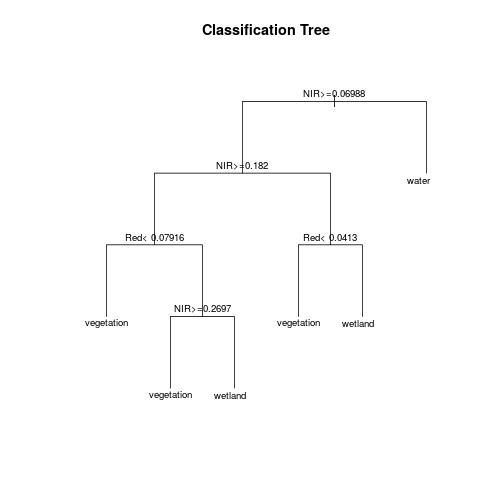Now predict the subset data based on the model. raster_out_filename_rp <- file.path(out_dir, paste0("r_predicted_rpart_", out_suffix, file_format)) r_predicted_rpart <- predict(r_stack, mod_rpart, type='class', filename = raster_out_filename_rp, progress = 'text', overwrite = T)  > plot(r_predicted_rpart)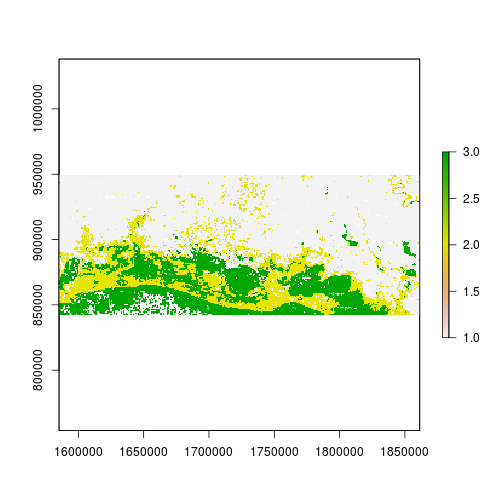r_predicted_rpart <- ratify(r_predicted_rpart) # creates a raster attribute table (rat) rat <- levels(r_predicted_rpart)[] rat$legend <- c("vegetation", "wetland", "water")
levels(r_predicted_rpart) <- rat

levelplot(r_predicted_rpart, maxpixels = 1e6,
col.regions = c("green","blue","darkblue"),
scales=list(draw=FALSE),
main = "Classification Tree")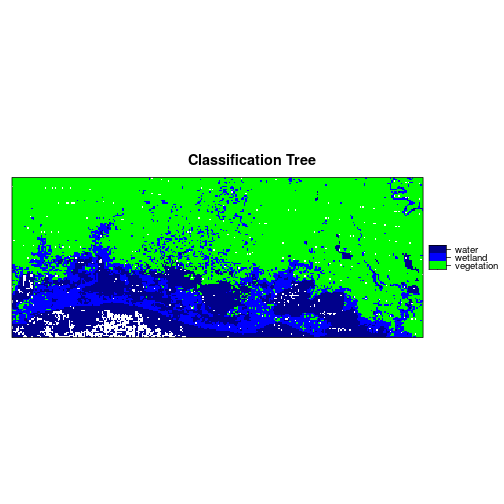### Support Vector Machine classification (SVM)

Set class_ID as factor to generate classification.

mod_svm <- svm(class_ID ~ Red + NIR + Blue + Green + SWIR1 + SWIR2 + SWIR3,
data=data_training, method="C-classification",

> summary(mod_svm)


Call:
svm(formula = class_ID ~ Red + NIR + Blue + Green + SWIR1 + SWIR2 +
SWIR3, data = data_training, method = "C-classification", kernel = "linear")

Parameters:
SVM-Type:  C-classification
SVM-Kernel:  linear
cost:  1

Number of Support Vectors:  111

( 11 55 45 )

Number of Classes:  3

Levels:
vegetation wetland water


Now predict the subset data based on the model.

raster_out_filename_sv <- file.path(out_dir, paste0("r_predicted_svm_", out_suffix, file_format))

r_predicted_svm <- predict(r_stack, mod_svm, progress = 'text',
filename = raster_out_filename_sv, overwrite = T)

plot(r_predicted_svm)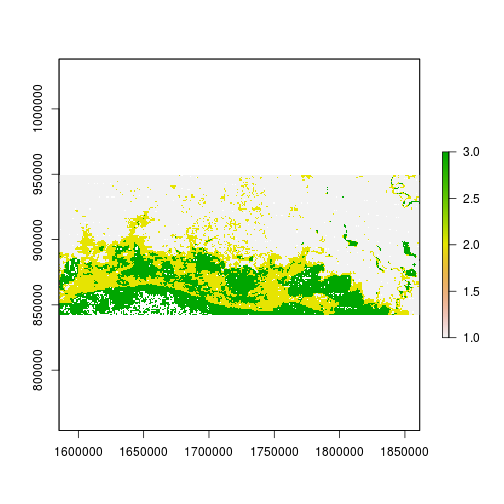rasterVis::histogram(r_predicted_svm)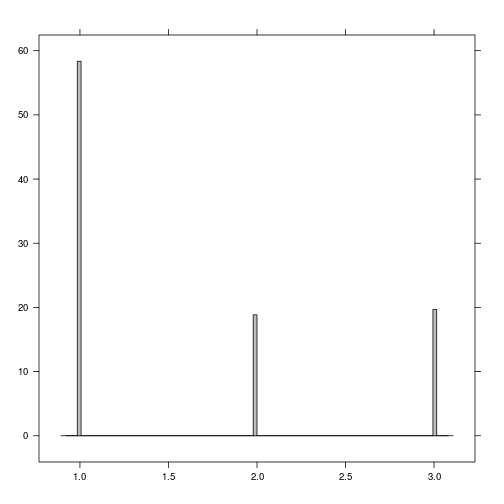r_predicted_svm <- ratify(r_predicted_svm)
rat <- levels(r_predicted_svm)[]
rat$legend <- c("vegetation", "wetland", "water") levels(r_predicted_svm) <- rat levelplot(r_predicted_svm, maxpixels = 1e6, col.regions = c("green","blue","darkblue"), scales = list(draw = FALSE), main = "SVM classification")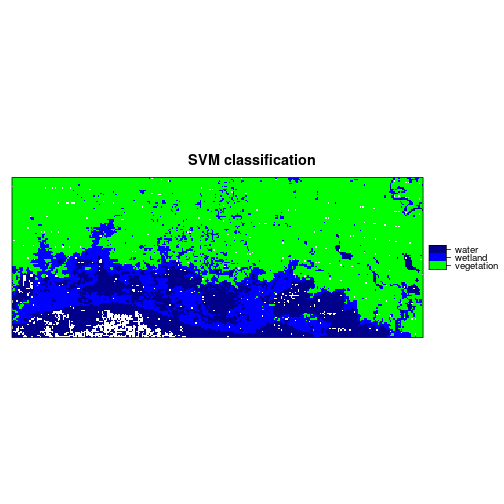## Part IV: Assessment and comparison of model performance Set up testing data: Full dataset, let’s use data points for testing. > dim(data_df)   1547 16  # omit values that contain NA, because may be problematic with SVM. data_testing <- na.omit(subset(data_df, training==0))  > dim(data_testing)   452 16  Predict on testing data using rpart model fitted with testing data. testing_rpart <- predict(mod_rpart, data_testing, type='class')  Predict on testing data using SVM model fitted with testing data. testing_svm <- predict(mod_svm, data_testing, type='class')  Predicted number of pixels in each class: table(testing_rpart)  testing_rpart vegetation wetland water 153 77 222  table(testing_svm)  testing_svm vegetation wetland water 152 84 216  ### Generate confusion matrix to assess the accuracy of the image classification More info on confusion matrices here: http://spatial-analyst.net/ILWIS/htm/ilwismen/confusion_matrix.htm Groundtruth data: > table(data_testing$class_ID)


vegetation    wetland      water
151         84        217


Classification model results:

> table(testing_rpart)

testing_rpart
vegetation    wetland      water
153         77        222

> table(testing_svm)

testing_svm
vegetation    wetland      water
152         84        216


To generate confusion matrix, need to use: testing_rpart: contains classification model prediction in the rows data_testing$class_ID: this column contains groundtruth data tb_rpart <- table(testing_rpart, data_testing$class_ID)
tb_svm <- table(testing_svm, data_testing$class_ID)  Matrices identifying correct and incorrect classifications by models: > tb_rpart   testing_rpart vegetation wetland water vegetation 148 5 0 wetland 3 70 4 water 0 9 213  > tb_svm   testing_svm vegetation wetland water vegetation 151 1 0 wetland 0 77 7 water 0 6 210  Accuracy (producer’s accuracy): the fraction of correctly classified pixels compared to all pixels of that groundtruth class. tb_rpart/sum(tb_rpart[,1]) # producer accuracy   0.9801325  tb_svm/sum(tb_svm[,1]) # producer accuracy   1  Looking at accuracy more closely: # overall accuracy for rpart sum(diag(tb_rpart))/sum(table(testing_rpart))   0.9535398  # overall accuracy for svm sum(diag(tb_svm))/sum(table(testing_svm))   0.9690265  Generate more accurate measurements from functions in the caret package: accuracy_info_rpart <- confusionMatrix(testing_rpart, data_testing$class_ID, positive = NULL)
accuracy_info_svm <- confusionMatrix(testing_svm, data_testing$class_ID, positive = NULL)  Overall accuracy produced: > accuracy_info_rpart$overall

      Accuracy          Kappa  AccuracyLower  AccuracyUpper   AccuracyNull
9.535398e-01   9.249994e-01   9.298553e-01   9.710138e-01   4.800885e-01
AccuracyPValue  McnemarPValue
3.536418e-108            NaN

> accuracy_info_svm$overall   Accuracy Kappa AccuracyLower AccuracyUpper AccuracyNull 9.690265e-01 9.503390e-01 9.485775e-01 9.829652e-01 4.800885e-01 AccuracyPValue McnemarPValue 3.961900e-118 NaN  Write out the results: table_out_filename <- file.path(out_dir, "confusion_matrix_rpart.txt") write.table(accuracy_info_rpart$table, table_out_filename, sep=",")

table_out_filename <- file.path(out_dir, "confusion_matrix_svm.txt")
write.table(accuracy_info_svm\$table, table_out_filename, sep=",")


Top of Section

If you need to catch-up before a section of code will work, just squish it's 🍅 to copy code above it into your clipboard. Then paste into your interpreter's console, run, and you'll be ready to start in on that section. Code copied by both 🍅 and 📋 will also appear below, where you can edit first, and then copy, paste, and run again.

# Nothing here yet!`